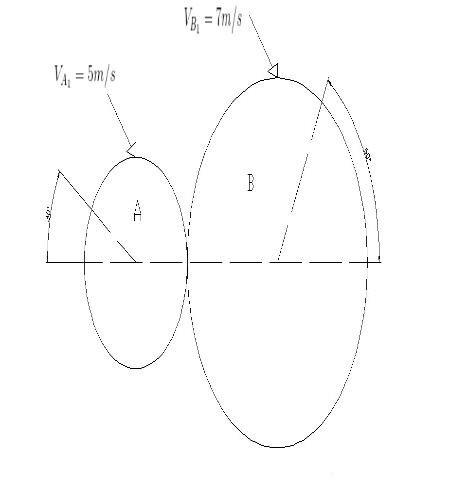Two discs A and B having masses 5 kg and 8 kg respectively collide in a smooth horizontal plane...

Question:

Two discs A and B having masses 5 kg and 8 kg respectively collide in a smooth horizontal plane along the directions shown. The coefficient of restitution is e = 0.65.

(a) Determine the velocities and of the particles after the impact and their directions.

(b) Determine the total energy loss as a result of the collision

(c) What does the energy loss represent?Conservation of energy

Energy of the system at initial condition is equal to energy of the final condition of the system. The conservation of energy can be of different type such as conservation of momentum, conservation angular momentum.

Given data:

• The mass of the disc A is: {eq}{m_A} = 5\;{\rm{kg}} {/eq}
• The mass of the disc B is: {eq}{m_B} = 7\;{\rm{kg}} {/eq}
• The coefficient of the restitution is: {eq}e = 0.65 {/eq}

The expression for the velocity component in horizontal and vertical directions,

{eq}\begin{align*} {V_{AH}} &= 5\cos 30^\circ = 4.33\;{\rm{m/s}}\\ {V_{AV}} &= 5\sin 30^\circ = 2.5\;{\rm{m/s}}\\ {V_{BH}} &= 7\cos 60^\circ = {\rm{3}}{\rm{.5}}\;{\rm{m/s}}\\ {V_{BV}} &= 7\sin 60^\circ = 6.06\;m/s \end{align*} {/eq}

a)

The expression for the conservation of the momentum in horizontal direction,

{eq}{m_{discA}}{V_{AH1}} + {m_{discB}}{V_{BH1}} = {m_{discA}}{V_{AH2}} + {m_{discB}}{V_{BH2}} {/eq}

Substitute the given data in the above expression,

{eq}\begin{align*} \left( {5 \times 4.23} \right) + \left( 8 \right)\left( { - 3.5} \right) &= 5{V_{AH2}} + 8{V_{BH2}}\\ 5{V_{AH2}} + 8{V_{BH2}} &= - 6.85......(I) \end{align*} {/eq}

The expression for the coefficient of restitution,

{eq}e = \dfrac{{{V_{BH2}} - {V_{AH2}}}}{{{V_{AH1}} - {V_{BH1}}}} {/eq}

Substitute the given data and equation (I) in the above expression,

{eq}\begin{align*} 0.65 &= \dfrac{{{V_{BH2}} - \left( { - 1.37 - 1.6{V_{BH2}}} \right)}}{{4.33 - \left( { - 3.5} \right)}}\\ {V_{BH2}} &= 1.469\;{\rm{m/s}} \end{align*} {/eq}

Substitute the final velocity of the disc B in horizontal direction into equation (I),

{eq}\begin{align*} {V_{AH2}} &= - 1.37 - 1.6\left( {1.469} \right)\\ & = 3.72{\rm{04}}\;{\rm{m/s}} \end{align*} {/eq}

The expression for the conservation of the moment in vertical direction,

{eq}{m_{discA}}{V_{AV1}} + {m_{discB}}{V_{BV1}} = {m_{discA}}{V_{AV2}} + {m_{discB}}{V_{BV2}} {/eq}

Substitute the given data in the above expression,

{eq}\begin{align*} \left( 5 \right)\left( {2.5} \right) + \left( 8 \right)\left( {6.06} \right) &= \left( 5 \right){V_{AV2}} + \left( 8 \right){V_{BV2}}\\ {V_{AV2}} &= 12.196 - 1.6{V_{BV2}}......(II) \end{align*} {/eq}

The expression for the coefficient of restitution,

{eq}e = \dfrac{{{V_{BV2}} - {V_{AV2}}}}{{{V_{AV1}} - {V_{BV1}}}} {/eq}

Substitute the given data and equation (II) in the above expression,

{eq}\begin{align*} 0.65 &= \dfrac{{{V_{BV2}} - \left( {12.196 - 1.6{V_{BV2}}} \right)}}{{2.5 - 6.06}}\\ {V_{BV2}} &= 4.077\;{\rm{m/s}} \end{align*} {/eq}

Substitute the final velocity of the disc A in vertical direction into equation (II),

{eq}\begin{align*} {V_{AV2}} &= 12.196 - 1.6\left( {4.077} \right)\\ &= 5.67\;{\rm{m/s}} \end{align*} {/eq}

The expression for the final velocity of disc A,

{eq}{V_{A2}} = \sqrt {{V^2}_{AV2} + {V^2}_{AH2}} {/eq}

Substitute the given data in the above expression,

{eq}\begin{align*} {V_{A2}} &= \sqrt {{{\left( { - 3.6} \right)}^2} + {{\left( {5.67} \right)}^2}} \\ &= 6.72\;{\rm{m/s}} \end{align*} {/eq}

Thus the final velocity of the disc A is {eq}6.72\;{\rm{m/s}} {/eq}

The expression for the final velocity of disc B,

{eq}{V_{B2}} = \sqrt {{V^2}_{BV2} + {V^2}_{BH2}} {/eq}

Substitute the given data in the above expression,

{eq}\begin{align*} {V_{B2}} &= \sqrt {{{\left( {1.469} \right)}^2} + {{\left( {4.077} \right)}^2}} \\ &= 4.33\;{\rm{m/s}} \end{align*} {/eq}

Thus the final velocity of the disc B is {eq}4.33\;{\rm{m/s}} {/eq}

The expression for the kinetic energy of the discs before collision,

{eq}K.{E_{before}} = \dfrac{1}{2}{m_{discA}}{V^2}_{A1} + \dfrac{1}{2}{m_{discB}}{V^2}_{B1} {/eq}

Substitute the values in the above expression,

{eq}\begin{align*} K.{E_{before}} &= \dfrac{1}{2}\left( 5 \right){\left( 5 \right)^2} + \dfrac{1}{2}\left( 8 \right){\left( 7 \right)^2}\\ &= 258.5\;{\rm{J}} \end{align*} {/eq}

The expression for the conservation of momentum before and after collision,

{eq}\begin{align*} {m_{discA}}{V_{A1}} + {m_{discB}}{V_{B2}} &= \left( {{m_1} + {m_2}} \right){V_2}\\ {V_2} &= \dfrac{{{m_{discA}}{V_{A1}} + {m_{discB}}{V_{B2}}}}{{\left( {{m_1} + {m_2}} \right)}} \end{align*} {/eq}

Substitute the values in the above expression,

{eq}\begin{align*} {V_2} &= \dfrac{{\left( 5 \right)\left( 5 \right) + \left( 8 \right)\left( 7 \right)}}{{5 + 8}}\\ & = 6.23\;{\rm{m/s}} \end{align*} {/eq}

The expression for the kinetic energy of the discs after collision,

{eq}K.{E_{after}} = \dfrac{1}{2}\left( {{m_{discA}} + {m_{discB}}} \right){V^2}_2 {/eq}

Substitute the values in the above expression,

{eq}\begin{align*} K.{E_{after}} &= \dfrac{1}{2}\left( {5 + 8} \right){\left( {6.23} \right)^2}\\ &= 252.36\;{\rm{J}} \end{align*} {/eq}

The expression for the loss in kinetic energy,

{eq}K.{E_{loss}} = K.{E_{aftre}} - K.{E_{before}} {/eq}

Substitute the given data in the above expression,

{eq}\begin{align*} K.{E_{loss}} &= 258.5 - 252.36\\ &= 6.153\;{\rm{J}} \end{align*} {/eq}

Thus the loss in kinetic energy is {eq}6.153\;{\rm{J}} {/eq}

C)

The energy loss represents the loss of the energy of the disc after collision and in this case the energy loss is {eq}6.153\;{\rm{J}} {/eq}.